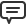# Blog

All Blog Posts  |  Next Post  |  Previous Post

#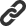New features in TMS Analytics & Physics 2.8

Bookmarks:

#### Tuesday, April 16, 2019

The previous version 2.7 of TMS Analytics & Physics library introduced the capability of converting formula and units of measurements to the TeX format. The TeX representation allows then drawing formula in natural math form, using some external tools, such as MathJax.
The new version 2.8 provides some improvements of formula conversion and includes new capabilities. The main new feature is converting standard functions to math operators. For example, exponent function ‘exp(x)’ can be converted to the form of power operation as ‘ex’. The following standard functions supported: sqrt, root, pow, abs and exp. The option of converting functions to the operation format can be on or off, allowing different representation of the formula.
Let us consider the following formula, containing several standard functions ‘sqrt(x^2-1)+root{3*n}(x/2)*abs(x-A)-exp(x^2)*pow{n/2}(x+1)’. This formula can be converted to the TeX format in two different ways: with or without converting functions to the operation format. Here is the formula drawing for these two cases:

• without function conversion: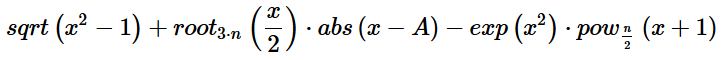• with function conversion: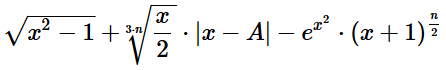Another improvement is new simplifications of math expressions. Simplifying math expressions allows better representation of math data. Simplified formula is more suitable for reading and perception. For example, the expression simplification implicitly used after symbolic derivative calculation, because this can significantly reduce the result. For example, let us consider the following formula: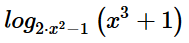Evaluating symbolic derivatives for the expression, we can get two results. The first result evaluated using formal recursive chain algorithm without simplifications: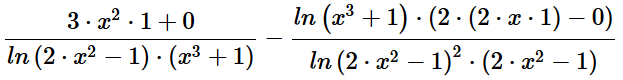Next formula is the same expression after simplification: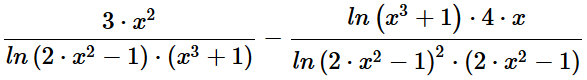The last version 2.8 of the library supports the following simplification algorithms:
• Expanding nested sum, product and power expressions
• Collecting constant addends in sum expressions
• Reducing addends in sum expressions
• Combining constant multipliers in product expressions
• Reducing multipliers in fraction expressions
• Combining power expressions with multipliers
• Simplifying integer square roots and modulus of negative data

The expression simplification can be used explicitly in the code, calling the ‘Simplify’ method of the ‘TTranslator’ class. Here is an example of the formula simplification demonstrating the algorithms: Initial formula: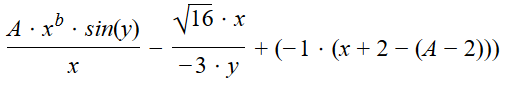Simplified formula: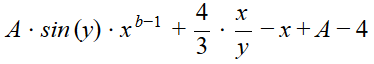TMS Analytics & Physics 2.8 is already available.

Masiha Zemarai

Bookmarks: